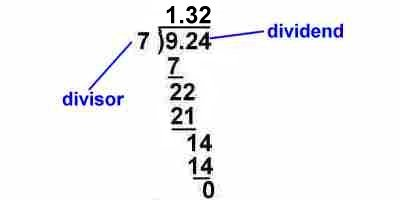Parents and Teachers: Support Ducksters by following us onor.

Kids Math

Multiplying and Dividing Decimals

Multiplying Decimals

If you already know how to multiply, then multiplying decimals will be easy, there is just one extra step you need to take.
• First, you multiply the numbers just like normal, as if the decimal point wasn't there.
• Next, you need to add in the decimal point to the answer. This is the only tricky part. You add up the decimal places there are in the numbers you multiplied. Then you put that many decimal places in the answer.
Let's try some examples:

1) 4.22 x 3.1 = ?

If you first just multiply 422 x 31 you get

 422 x 31 13082

Now, there are 2 decimal places in 4.22 and 1 decimal place in 3.1. This is a total of 3 decimal places. We then put three decimal places in 13082 and we finally get the answer:

13.082

1) 4.220 x 3.10 = ?

To show how the decimal part works, we'll solve the same problem again, but this time we will add a zero to the right of each number we multiply. This doesn't change the value of the numbers, so it shouldn't change the answer we get.

First we multiply without worrying about the decimals:

 4220 x 310 1308200

Now, we count the total amount of decimals. There are 5 total decimals places this time. If we count over 5 decimal places from the end of 1308200 we get the same answer:

13.08200

Note: the extra zeros to the right of the decimal point do not change the value of the number.

Dividing Decimals

When you are dividing a number with a decimal point by a whole number, dividing decimals is pretty simple.
• Divide the number as you normally would, using long division.
• Bring the decimal point directly up from the dividend.
Example:

9.24 ÷ 7 = ?If both divisor and dividend are decimals:

If the divisor is a decimal number rather than a whole number, then you need to take an extra step. In this step you convert the divisor from a decimal number to a whole number. You do this by moving the decimal point to the right in the divisor until there are no more numbers other than zero to the right of the decimal point. Then you move the decimal point to the right the same number of places in the dividend.

Example of moving the decimal point for division:

9.24 ÷ 7.008

You want the divisor 7.008 to be a whole number, so you need to move the decimal point 3 places to the right:

7008

Now you need to move the decimal point for the dividend 3 places to the right:

9240

In this case you have to add a zero to move it 3 places.

Now you divide 9240 by 7008 to get the answer:

Example:

0.64 ÷ 3.2 = ?

First move the decimal points so the divisor is a whole number:

6.4 ÷ 32 = ?Important things to remember:
• Move the decimal point directly up in your long division.
• Always move the decimal points on BOTH to divisor and the dividend to where the divisor is a whole number.

Back to Kids Math

Back to Kids StudyFollow us onor